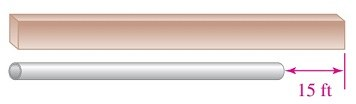# Exercise 2: Concepts

👁 69
statistics

Questions #: 5
Time: 10 minutes
Pass Score: 80.0%
Style
Mode

#### CUTLERY

The knife shown below is 12 inches long. Write an expression that represents the length of the bladePOINTS (1)

#### SAVINGS ACCOUNTS

A student inherited \$5,000 and deposits dollars in American Savings. Write an expression that represents the amount of money left to deposit in a City Mutual account

POINTS (1)

#### MIXING SOLUTIONS

Solution 1 is poured into solution 2. Write an expression that represents the number of ounces in the mixture

Solution 1 = 20 ounces

Solution 2 = x ounces

POINTS (1)

#### SNACKS

Cashews were mixed with p pounds of peanuts to make 100 pounds of a mixture.  Write expression that represents the number of pounds of cashews that were used.

POINTS (1)

#### BUILDING MATERIALS1. Let b = the length of the beam shown below (in feet). The expression that represents the length of the pipe is (1)2. Let p = the length of the pipe (in feet). The expression that represents the length of the beam is (2)drag and drop the selected option to the right place or type it instead
(b - 15) feet
(b + p) feet
(p + 15) feet
(b + p -15) feet
POINTS (1)